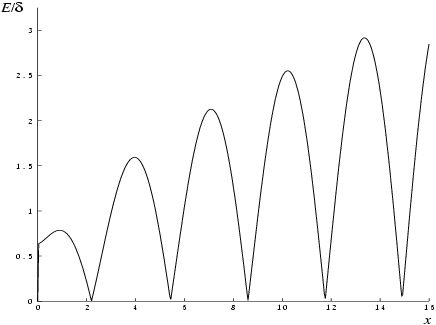NAG Library Function Document

1Purpose

nag_bessel_y0 (s17acc) returns the value of the Bessel function ${Y}_{0}\left(x\right)$.

2Specification

 #include #include
 double nag_bessel_y0 (double x, NagError *fail)

3Description

nag_bessel_y0 (s17acc) evaluates an approximation to the Bessel function of the second kind ${Y}_{0}\left(x\right)$.
Note:  ${Y}_{0}\left(x\right)$ is undefined for $x\le 0$ and the function will fail for such arguments.
The function is based on four Chebyshev expansions:
For $0,
 $Y0x=2π ln⁡x∑r=0′arTrt+∑r=0′brTrt, with ​t=2 x8 2-1.$
For $x>8$,
 $Y0x=2πx P0xsinx-π4+Q0xcosx-π4$
where ${P}_{0}\left(x\right)=\underset{r=0}{{\sum }^{\prime }}\phantom{\rule{0.25em}{0ex}}{c}_{r}{T}_{r}\left(t\right)$,
and ${Q}_{0}\left(x\right)=\frac{8}{x}\underset{r=0}{{\sum }^{\prime }}\phantom{\rule{0.25em}{0ex}}{d}_{r}{T}_{r}\left(t\right),\text{with ​}t=2{\left(\frac{8}{x}\right)}^{2}-1\text{.}$
For $x$ near zero, ${Y}_{0}\left(x\right)\simeq \frac{2}{\pi }\left(\mathrm{ln}\left(\frac{x}{2}\right)+\gamma \right)$, where $\gamma$ denotes Euler's constant. This approximation is used when $x$ is sufficiently small for the result to be correct to machine precision.
For very large $x$, it becomes impossible to provide results with any reasonable accuracy (see Section 7), hence the function fails. Such arguments contain insufficient information to determine the phase of oscillation of ${Y}_{0}\left(x\right)$; only the amplitude, $\sqrt{\frac{2}{\pi n}}$, can be determined and this is returned on failure. The range for which this occurs is roughly related to machine precision; the function will fail if  (see the Users' Note for your implementation for details).
Abramowitz M and Stegun I A (1972) Handbook of Mathematical Functions (3rd Edition) Dover Publications
Clenshaw C W (1962) Chebyshev Series for Mathematical Functions Mathematical tables HMSO

5Arguments

1:    $\mathbf{x}$doubleInput
On entry: the argument $x$ of the function.
Constraint: ${\mathbf{x}}>0.0$.
2:    $\mathbf{fail}$NagError *Input/Output
The NAG error argument (see Section 3.7 in How to Use the NAG Library and its Documentation).

6Error Indicators and Warnings

NE_ALLOC_FAIL
Dynamic memory allocation failed.
See Section 2.3.1.2 in How to Use the NAG Library and its Documentation for further information.
NE_INTERNAL_ERROR
An internal error has occurred in this function. Check the function call and any array sizes. If the call is correct then please contact NAG for assistance.
See Section 2.7.6 in How to Use the NAG Library and its Documentation for further information.
NE_NO_LICENCE
Your licence key may have expired or may not have been installed correctly.
See Section 2.7.5 in How to Use the NAG Library and its Documentation for further information.
NE_REAL_ARG_GT
On entry, ${\mathbf{x}}=〈\mathit{\text{value}}〉$.
Constraint: ${\mathbf{x}}\le 〈\mathit{\text{value}}〉$.
x is too large. On failure the function returns the amplitude of the ${Y}_{0}$ oscillation, $\sqrt{2/\left(\pi x\right)}$.
x is too large, the function returns the amplitude of the ${Y}_{0}$ oscillation, $\sqrt{\frac{2}{\pi x}}$.
NE_REAL_ARG_LE
On entry, ${\mathbf{x}}=〈\mathit{\text{value}}〉$.
Constraint: ${\mathbf{x}}>0.0$.
${Y}_{0}$ is undefined, the function returns zero.

7Accuracy

Let $\delta$ be the relative error in the argument and $E$ be the absolute error in the result. (Since ${Y}_{0}\left(x\right)$ oscillates about zero, absolute error and not relative error is significant, except for very small $x$.)
If $\delta$ is somewhat larger than the machine representation error (e.g., if $\delta$ is due to data errors etc.), then $E$ and $\delta$ are approximately related by
 $E≃xY1xδ$
(provided $E$ is also within machine bounds). Figure 1 displays the behaviour of the amplification factor $\left|x{Y}_{1}\left(x\right)\right|$.
However, if $\delta$ is of the same order as the machine representation errors, then rounding errors could make $E$ slightly larger than the above relation predicts.
For very small $x$, the errors are essentially independent of $\delta$ and the function should provide relative accuracy bounded by the machine precision.
For very large $x$, the above relation ceases to apply. In this region, ${Y}_{0}\left(x\right)\simeq \sqrt{\frac{2}{\pi x}}\mathrm{sin}\left(x-\frac{\pi }{4}\right)$. The amplitude $\sqrt{\frac{2}{\pi x}}$ can be calculated with reasonable accuracy for all $x$, but $\mathrm{sin}\left(x-\frac{\pi }{4}\right)$ cannot. If $x-\frac{\pi }{4}$ is written as $2N\pi +\theta$ where $N$ is an integer and $0\le \theta <2\pi$, then $\mathrm{sin}\left(x-\frac{\pi }{4}\right)$ is determined by $\theta$ only. If $x\gtrsim {\delta }^{-1}$, $\theta$ cannot be determined with any accuracy at all. Thus if $x$ is greater than, or of the order of the inverse of machine precision, it is impossible to calculate the phase of ${Y}_{0}\left(x\right)$ and the function must fail.Figure 1

8Parallelism and Performance

nag_bessel_y0 (s17acc) is not threaded in any implementation.

None.

10Example

This example reads values of the argument $x$ from a file, evaluates the function at each value of $x$ and prints the results.

10.1Program Text

Program Text (s17acce.c)

10.2Program Data

Program Data (s17acce.d)

10.3Program Results

Program Results (s17acce.r)

© The Numerical Algorithms Group Ltd, Oxford, UK. 2017